These Pages include various standards. To confirm the status of any standard, identify the replacement standard if it is obsolete and/or purchase the standard please use. BSI Shop It is also possible to become a BSI member and obtain copies of the Standards at much reduced prices.

Keyways Index

Key /Spline Strength Calculations

Introduction..... Types of coupling...... Shaft strength...... Keys...... Straight Spline...... Involute Spline...... Example Calculations......

Introduction

The following notes indicate how to calculate a reasonable value for the stress resulting from an applied torque. The calculation includes adjustment for the operating conditions by including a Service factor Ks . This factor is based on a design factor Kd, an application factor Ka , a load distribution factor Km, a fatigue life factor Kf, and a wear life factor Kw.    The latter factor primarily related to splines.

This working stress should be such that it is less than the Material Design strength / Fs (Safety Factor ).

I have found that the Mitcalc.com excel based calculation sheets (connections) see link 1 below provides a very convenient ( and more accurate ) method of completing these calculations

Machinerys Handbook (27th ed.) includes tables for allowable stresses for splines.    The allowable compressive stresses indicated are very low compared to the stresses indicated on this site (10%).    The links below to the Zakgear Calculator and Wikipedia also show the same tables.     These documents are based of the article �When Splines Need Stress Control� by Darle W. Dudley".    The different calculation methods should result in the same Safety Factors if the same interpretation of a fixed and a flexible coupling is used and if the design factor Kd is correctly applied The method used by Dudley involves use of a separate equation for a flexible coupling and fixed coupling and does not use a factor

I have completed the example calculation on this page using the Zakgear calculator using imperial equivalents to my metric values. The resulting margins of safety (FofS-1)relate very closely to my Factors of safety.

Note: Tables of the various factors and design strengths are found on webpage. Factors.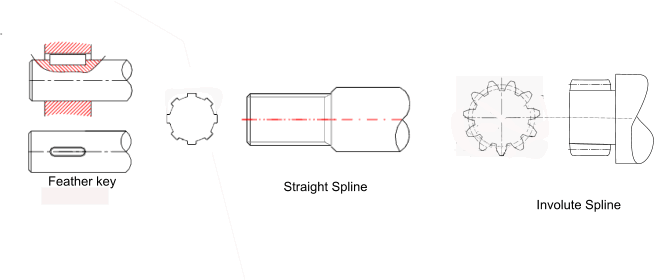Shaft Capacity

It is first good practice to estimate the torque which can be transmitted by the reduced shaft diameter i.e the diameter of the shaft which is not including the depth of the key /spline.

Note: Tables of the various factors and design strengths are found on webpage. Factors.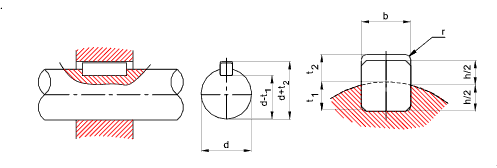Do = Shaft diameter(m) Di = Reduced diameter (m) Dh = Hole diameter- Hollow shaft (m) T = Applied torque (N.m) K s = Service factor....for shafts K s = K a/ K f σc = Resulting Compressive stress of key (N/m2) τ = Resulting Shear stress in key (N/m2)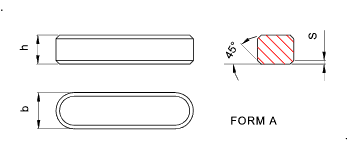Key Capacity

Notes: Tables of the various factors and design strengths are found on webpage. Factors.

To determine the torque capacity of key..

 x = Depth of keyface (key/shaft) taking force (m) (see sketch) x1 = Depth of keyface (key/hub) taking force (m) (see sketch) r = Radius of shaft = Do/2 (m) Le = Effective Length of key = Straight Length (m) b = Breadth depth of key (m) c = Key Chamfer size (m) t1 = Depth of keyway (m) T = Applied Torque (N.m ) F = Force on key = T/r (approx.) (N ) Ks = Service factor..       For a fixed / close fit keyway K s = K a . K d / K f       For a sliding fit keyway K s = K a . K d / K w σc = Resulting Compressive stress of key (N/m2) τ = Resulting Shear stress in key (N/m2) It is reasonable to use x = h/2 however the sketch below provides a more accurate value of x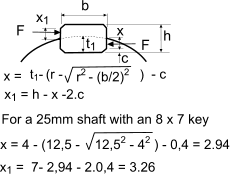Note: The stress in the hub need only be considered if the hub material design strength is significantly lower than the shaft or key strength.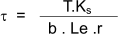based on shear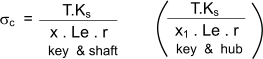based on compressive strength

ISO straight sided Spline Capacity

Notes: Tables of the various factors and design strengths are found on webpage. Factors.

 r = Mean radius of spline (m) n = Number of splines Le = Effective Length of spline = Straight Length (m) d = Depth of Spline (m) T = Applied Torque (N.m ) Ks = Service factor..       For a fixed /Guided spline K s = K a. . K d / K f       For a flexible / sliding fit spline K s =K a . K m . K d / K w σc = Resulting Compressive stress in spline (N/m2) τ = Resulting Shear stress in spline (N/m2)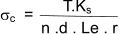based on compressive strength

ISO straight involute Spline Capacity

Notes: Tables of the various factors and design strengths are found on webpage. Factors.

 D = Pitch dia of spline (m) m = spline module (m) S = Tooth thickness (m) z = Number of teeth Le = Effective Length of spline = Straight Length (m) h = Depth of engagement of spline teeth T = Applied Toque (N.m ) Ks = Service factor..       For a fixed close fit /Guided spline K s = K a / K f       For a flexible / sliding fit spline K s =K a . K m . K d / K w sc = resulting compressive stress in shaft material (N/m2) t = resulting shear stress in shaft material (N/m2) Note: h for for 30o flat root splines h = 0,9m and for fillet root splines h = m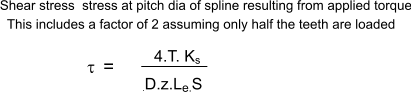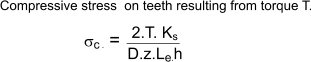Example calculations

Three example calculations are provided below all based on the same transmitted power and RPM .    The keys and splines are all assumed to be close fits and guided.    I have obtained the various factors and material strengths using the tables on webpage Factors    These calculations are also completed using Mitcalc comparing the approximate methods as indicated on this page with more accurate methods .

I have simply identified a transmitted power 10 kW. and by a process of iteration arrived at a reasonable shaft diameter (25mm) . I have then calculated the necessary key/spline proportions to transmit the power with a factor of safety about 2,5. In the calculations I have shown the formula but not the detailed numbers and unit conversions.

Initial data

 Power transferred 10 kw RPM = 1500 Resulting torque = P/(n.9549) = 63,66 Nm Design life = 10,000 hours Start Stops = 100,000 hours Total revolutions of shaft = 900x 106 revs. Shaft /Hub/keyway material (BS EN 10083 C45 normalised. Ultimate tensile strength 580MPa, Yield stress approx 300 MPa based on the table on webpage Factors. I've selected a shear strength = Ss = 200 MPa, and a compressive strength = Sc = 130 MPa

Factors

 Design Factor Kd = 1.0 Application Factor Ka = 1.0 Load Distribution Factor Km = 1.0 Fatigue Factor Kf = 0.5 Wear Factor Kw = 0.7 Service Factor Shaft Ks = 2.0 Service Factor key (close fit) Kk = 2.0 Service Factor spline (close fit) Ksp = 2.0

Shaft Calculation

Do : Assuming a basic shaft size of 25mm .

The shear stress in the shaft is calculated as

τ = 16.T . Ks / (π.Do3 ) =41,5 MPa (F of Safety (Ss /τ ) of about 4,8)

Key Calculation

A key of 8 mmx 7mm by 70mm long is selected.

Le The effective length of the key = 70-2*4 = 62mm =
x (calculated above = 2,94mm,
x1 (Calculated above = 3,26mm )
Di Reduced shaft dia = 25mm - (t1 = 4) = 21mm

The shear stress in the reduced shaft dia (Di) =

τ = 16.T . Ks / (π.Di3 ) = 16 . 63,67 . 1000. 2 / (π . 213 . ) = 70,02 MPa ........ (F of Safety (Ss /τ ) of about 2 ,8)

using Mitcalc σc = 70 MPa

The compressive stress in the key and the shaft. This is the worst case stress in this example

σc = T . Ks /( Le.(D/2).x) = 63,67 . 1000 . 2 . 2 / (62 . 25. 2,94 ) = 55,83 MPa .... (F of Safety (Sc /σ ) of about 2,33)

using Mitcalc σc = 58,5 MPa

Straight Spline Calculation

A straight spline of 25 - 6x21x25 is selected. This has a OD of 25 mm
Di reduced dia= 21 mm
n = 6 teeth
Le: The length of spline selected = 25mm .
Di: The reduced diameter = 21mm,
r: the mean readius of the teeth = (21+25)/4 = 11,5mm
c: The chamfer radius on the teeth (top and bottom) is assumed to be about c = 0,3mm.
y: The resulting effective depth of the teeth (y) = ((D- Di)/2 -2.c = 1,4mm

The shear stress in the reduced shaft dia (Di) =

τ = 16.T . Ks / (π.Di3 ) = 16 . 63,67 . 1000 . 2 /( π . 213 ) = 70,02 MPa ........ (F of Safety (Ss /τ )of about 2 ,8)

using Mitcalc σc = 70 MPa

The compressive stress in the spline and the shaft. This is the worst case stress in this example

σc = T . Ks /( Le.n.r.y) = 63,67 . 1000 . 2 /( 25 . 6 . 11,5 . 1,4 ) = 52,72 MPa .... (F of Safety (Sc /σ c ) of about 2,47)

using Mitcalc σc = 52,7 MPa

Involute Spline Calculation

An involute spline of 25 - 1 x 24 is selected. This has a OD (Dee ) of 25 mm a PCD ( D ) = 24mm , a module (m) of 1, Number of teeth z = 24

z : Number of teeth = 24
L: The length of spline selected = 10mm
D : Pitch diameter = 24mm = m.z
Die: The reduced diameter = 22,5mm = m(z-1,5)
Dee: Outside Diameter = 25mm = m(z+1)
L: Length = 10mm (estimated)
p: Pitch = 3,1416mm = m.π
t: Tooth thickness = 1,157mm = p/2
h : Tooth height =0,9m = 0,9mm

The chamfer radius (c) on the teeth is assumed to be about c = 0,3mm. Th resulting effective depth of the teeth (y) = ((D- Di)/2 -2.h = 1,4mm

The shear stress in the reduced shaft dia (Di) =

τ = 16.T . Ks / (π.Die3 ) = 16 . 63,67 . 1000 . 2 / (π . 22,53 ) = 56,9 MPa.... (F of Safety (Ss /τ )of about 3,51)

using Mitcalc τ = 56,9 MPa

The shear stress in the spline teeth.

τ = 4 . T . Ks /(L.z.t.D) = 2. 63,67 . 1000 . 2 /( 10 . 24 . 1,57 . 24 ) = 56,28 MPa ..... (F of Safety (Ss /σ ) of about 3,55)

The compressive in the spline teeth.

σc = 2 .T . Ks /(L.z.h.D) = 2 . 63,67 . 1000 . 2 /( 10 . 24 . 0,9 . 24 ) = 49,12 MPa ..... (F of Safety (Sc /σc; ) of about 2,65)

using Mitcalc σc = 46,3 MPa

 Sites Providing Relevant Information Mitcalc.com...Excel based programme allowing detailed design of shaft connection (19 Eur) Zakgear-Spline Stress Calculation... Calculator and associated equations of tables - Extemely useful - US units (not metric) Ondrives...Supplier of drive components including keys and splines
Keyways Index